# canvas 动画真好玩，快来学一下这炫酷的效果吧！### 前言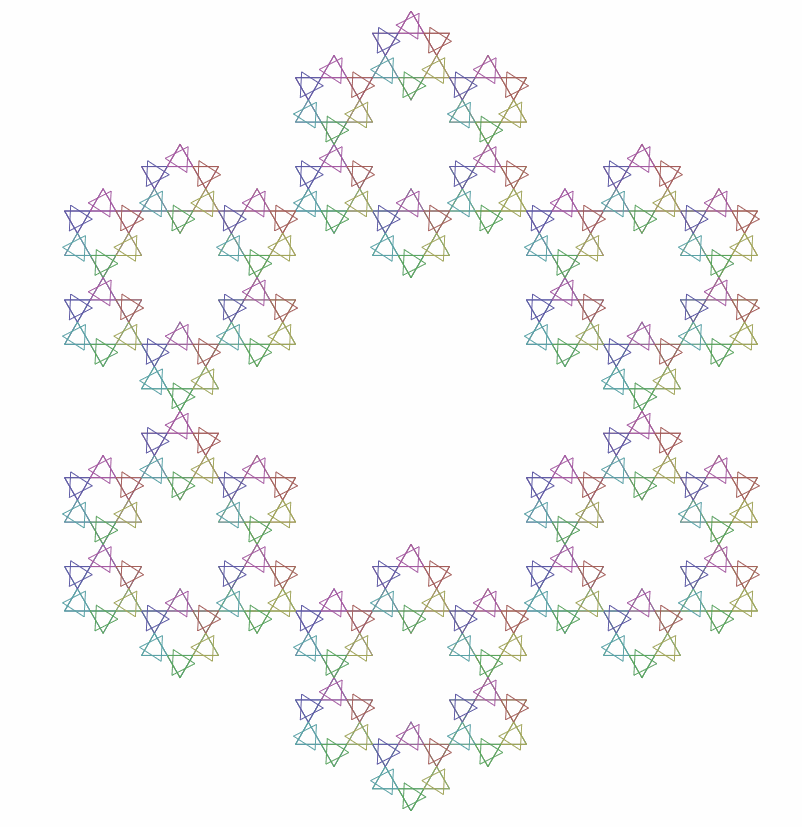### 旋转的大三角形

``````class RotationTriangle {
constructor() {
/** @type {HTMLCanvasElement} */
this.canvas = document.getElementById('canvas');
this.ctx = this.canvas.getContext('2d');
this.canvas.width = 800;
this.canvas.height = 800;
this.tris = [];  // 保存所有的三角形对象
}
}

``````class Triangle {
constructor(x, y, r, d, ctx) {
this.x = x; // 三角形中心的x坐标
this.y = y; // 三角形中心的y坐标
this.r = r; // 中心到顶点的距离
this.d = d; // 初始角度
this.td = this.d; // 旋转角度
this.ctx = ctx;
}
// 更新方法
update() {
// 增加旋转角度
this.td++;
}
// 绘制方法
draw() {
this.drawTri(this.x, this.y, this.r, this.d);
this.drawTri(this.x, this.y, this.r, this.td);
}
// 绘制三角形
drawTri(x, y, r, d) {
this.ctx.beginPath();
for (let i = 0; i < 3; i++) {
const { x: x1, y: y1 } = this.getCoordinate(x, y, r, d + i * 120);
this.ctx.lineTo(x1, y1);
}
this.ctx.closePath();
this.ctx.stroke();
}
// 根据三角函数计算出对应的坐标点
getCoordinate(x, y, r, d) {
let x1 = r * Math.cos(d * Math.PI / 180) + x;
let y1 = r * Math.sin(d * Math.PI / 180) + y;
return { x: x1, y: y1 };
}
}

`Triangle` 类主要是用于生成三角形，其中我们需要传入五个参数，这五个参数在代码中已经添加了相关的注释。接下来我们就需要在 `RotationTriangle` 类中通过实例化 `Triangle` 类来渲染外部的三角形，并让它们旋转起来，我们一起来给 `RotationTriangle` 类添加几个方法，相关代码如下：

``````class RotationTriangle {
constructor() {
...other code

this.init();
this.animate();
}
init() {
this.tris.push(
new Triangle(this.canvas.width / 2, this.canvas.height / 2, this.canvas.width / 2, -90, this.ctx)
);
}
draw() {
for (const n in this.tris) {
const tris = this.tris[n];
tris.update();
tris.draw();
}
}
animate() {
requestAnimationFrame(() => this.animate());
this.draw();
}
}

new RotationTriangle();

`RotationTriangle` 类中，我们添加了一个 `init` 方法，主要用于初始化生成的三角形，最后不要忘了实例化 `RotationTriangle` 类，实现的效果如下图所示：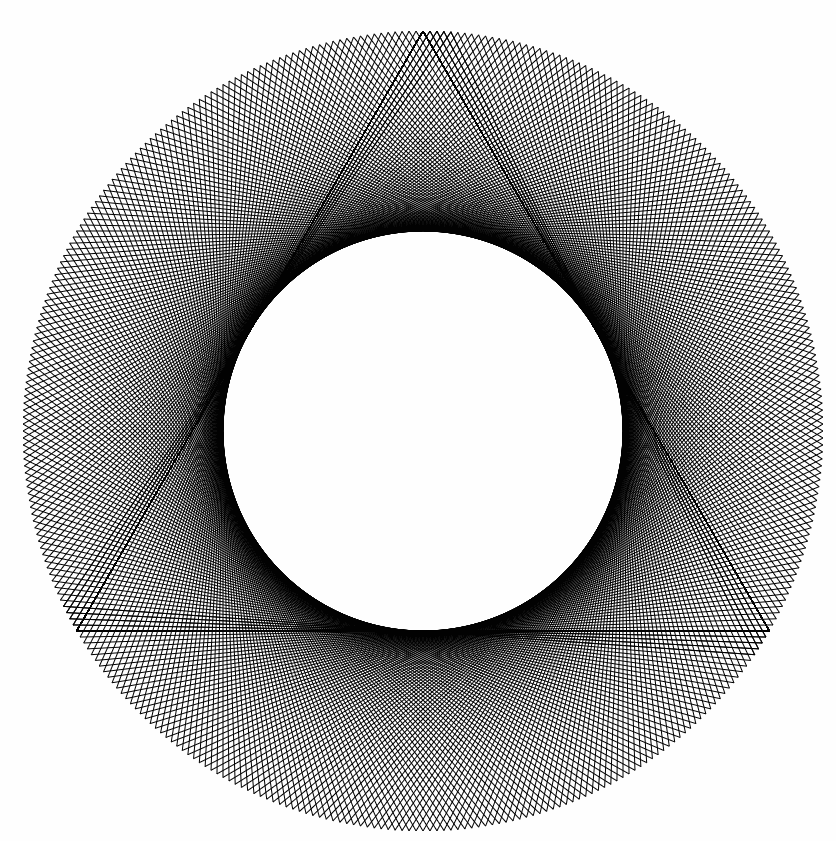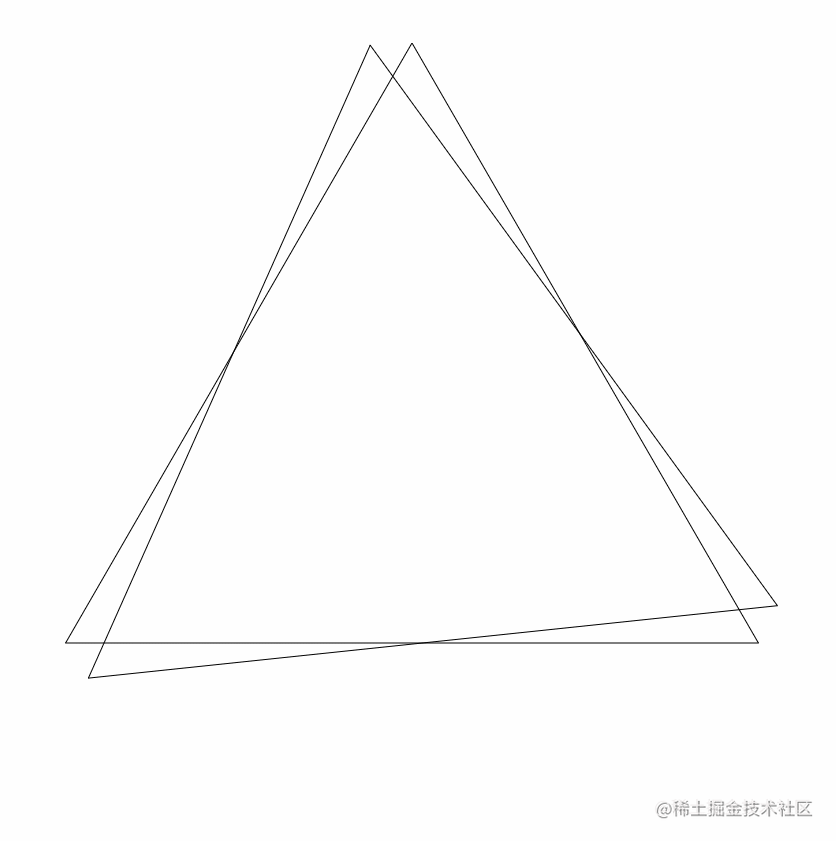`Triangle` 类的 `update` 方法中，我们通过 `this.td++` 不断的改变旋转角度，然后执行 `this.draw` 方法来绘制三角形，就实现了三角形的旋转。那么我们该如何让这个旋转的三角形到达一定角度后就停下来呢？其实也很简单，我们只需要修改 `Triangle` 类中的 `update` 方法即可，让我们一起来看一下修改后的代码，如下：

``````class Triangle {
...other code
update() {
// 限制旋转角度
if (this.td < this.d + 60) {
// 增加旋转角度
this.td++;
}
}
...other code
}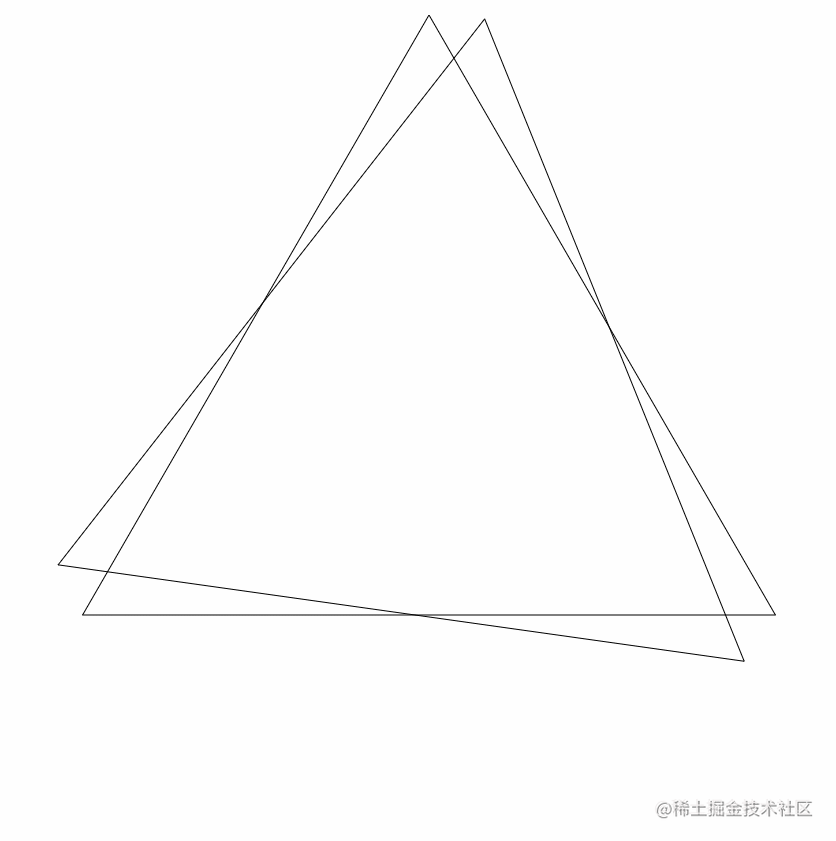### 旋转的小三角

``````class RotationTriangle {
...other code

draw() {
this.ctx.clearRect(0, 0, this.canvas.width, this.canvas.height);
for (const n in this.tris) {
const tris = this.tris[n];
tris.update();
tris.draw();

// 渲染两个大三角形的六个顶点周围的小三角形
// 首先需要判断一下当前大三角形的旋转是否已经停止了
if (tris.td === tris.d + 60) {
// 因为是两个大三角形，因此有6个顶点
for (let m = 0; m < 6; m++) {
// 根据三角函数，小三角的中心到外部三角的中心距离相当于外部三角距离顶点的2/3
const nr = tris.r * 2 / 3;
// 新的三角形的旋转角度，默认为外部三角形的角度
const d = tris.d + m * 60;
// 计算坐标，根据外部三角行的角度旋转六次，即可获得新的三角形中心的坐标
const x = nr * Math.cos(d * Math.PI / 180) + tris.x;
const y = nr * Math.sin(d * Math.PI / 180) + tris.y;
// 计算新的三角形的顶点，距离相当于外部三角形距离顶点的1/3
const r = tris.r / 3;
this.tris.push(new Triangle(x, y, r, d, this.ctx));
}
}
}
}
}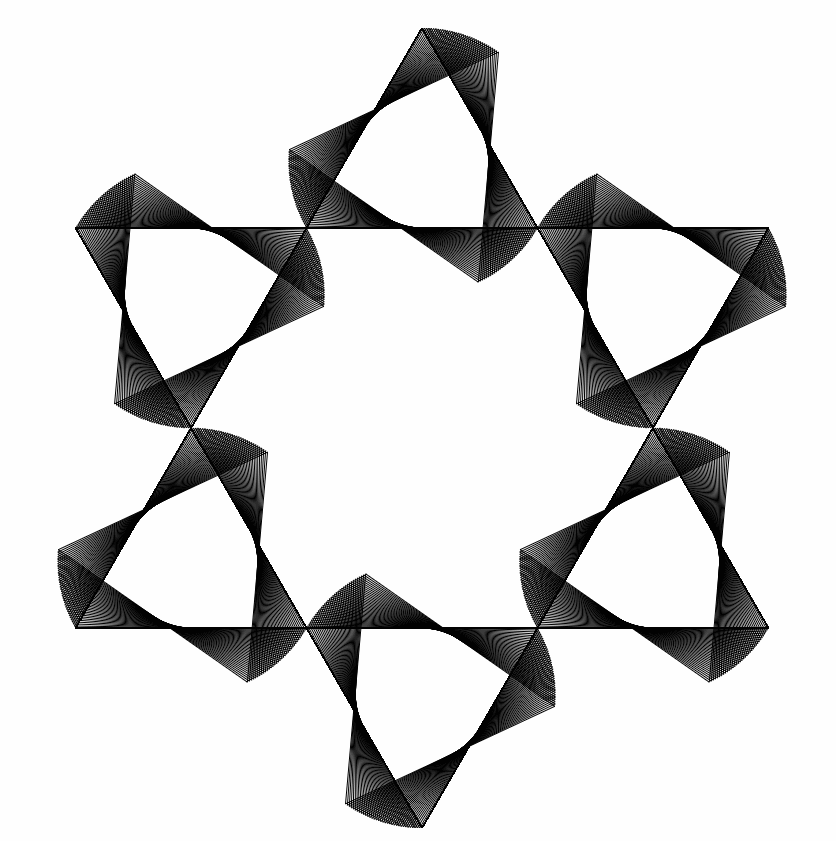``````class Triangle {
constructor(x, y, r, d, ctx) {
...other code
// 添加一个开关即可
this.act = true; // 旋转开关，当旋转到指定的位置后，才开始生成新的小三角形
}
}

`Triangle` 类中有了旋转的开关后，我们就需要修改一下 `RotationTriangle` 类中的 `draw` 方法内的判断条件了，相关代码如下：

``````class RotationTriangle {
... other code
draw() {
... other code

// 判断一下当前大三角是否旋转到了指定的角度，并且需要限制一下生成的三角形尺寸，不能无限制的生成下去
if (tris.td === tris.d + 60 && tris.r > 10 && tris.act) {
...other code

// 开关设置为false，停止旋转
tris.act = false;
}
}
}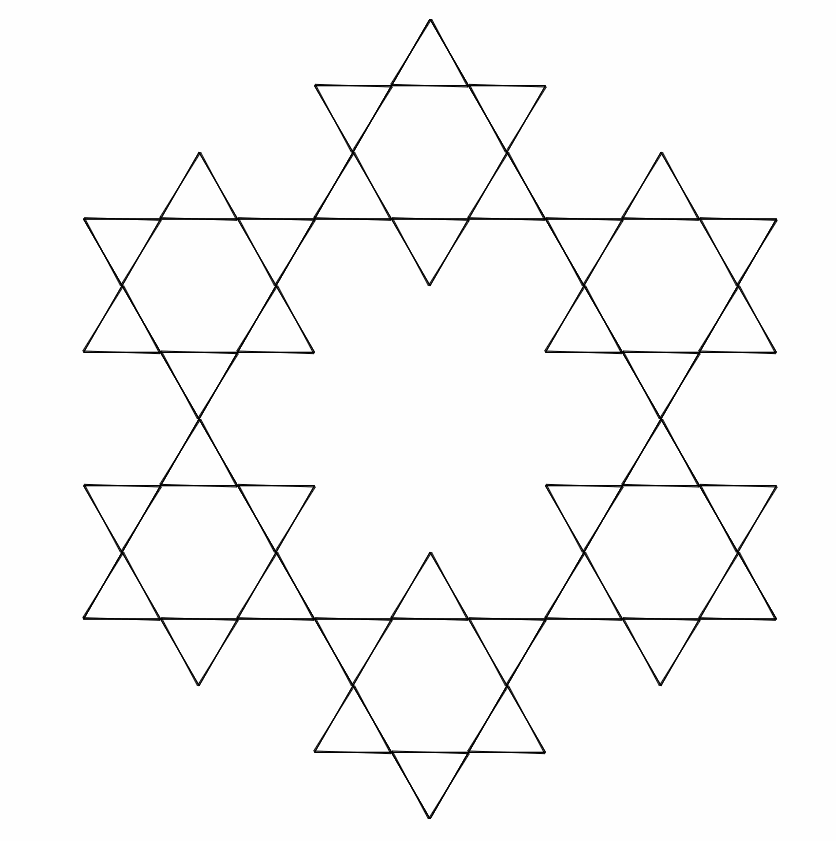### 炫丽的旋转三角形

``````class Triangle {
constructor(x, y, r, d, ctx) {
...other code

// 旋转180度后，“清空” canvas
this.opt = 180; // 当旋转到对应角度时，画布中的三角形淡出画布
}
...other code

drawTri(x, y, r, d) {
this.ctx.beginPath();
for (let i = 0; i < 3; i++) {
const { x: x1, y: y1 } = this.getCoordinate(x, y, r, d + i * 120);
this.ctx.lineTo(x1, y1);
// 添加 strokeStyle 即可，颜色值属性为 hsl
this.ctx.strokeStyle = `hsl(\${this.td}deg, 30%, 50%, \${this.opt / 60})`;
}
this.ctx.closePath();
this.ctx.stroke();
}
}

`Triangle` 类的 `drawTri` 方法中，我们添加了 `strokeStyle` 属性值，并且使用 `hsl` 作为颜色的变换属性，如果不了解 `hsl` 相关的参数，可以点击这里进行查看。`hsl` 前三个参数分别就对于了颜色的色调值饱和度以及颜色的亮度，而最后一个参数则代表当前的透明度，当我们在前面定义的 `this.opt` 遍历值除以 60 后，`canvas` 中的三角形就会逐渐淡出画布，看起来就像被“清除”了一样。

``````class RotationTriangle {
constructor() {
...other code
this.count = 0;

this.init();
}
init() {
this.count = 480;
...other code
}
draw() {
...other code

this.count--;
if (this.count < 0) {
this.tris.length = 0;
this.init();
}
}
}

`draw` 方法中，通过不断的减小 `this.count` 的值，当它小于 0 时，我们就将 `this.tris` 数组的长度重置为 0，这样就减少 `canvas` 绘制时浏览器内存的消耗；其次我们重新执行 `this.init()` 方法，这样就能够让整个效果无限的执行下去了，最终完整的代码及执行效果可以在这里进行查看：